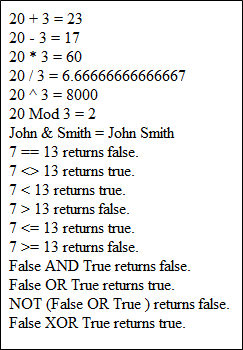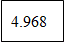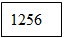# VBScript Operators: Logical (AND, OR) Arithmetic, Comparison Example

## VBScript Operators

An Operator works either on values or variables to perform some task. Operators are very crucial in programming because you cannot assign values to variables or perform tasks without them.

Suppose, you want to calculate the sum of two variables a and b and save the result in another variable c.

`c = a + b`

Here, a, b and c are operands and + and = are the operators.You might be familiar with the first four arithmetic operators as we use them commonly.

The modulus operator is used to find the remainder after a division. For example, 10%3 is equal to 1.

The exponentiation operator is equivalent to “the power of” in mathematics. For example, 2^3 is equal to 8.

The concatenation operator is used to concatenate two string values.

For example, “Hello” & ” John” will return “Hello John”.

### VBScript Comparison Operators

Comparison operators are used to compare two values.

Different comparison operators are == , <>, < , >, <= and >=. Suppose, you have two variables a and b with values 5 and 9 respectively, then the results for the following comparison will be like this:

```a==b  will return false.
a<>b will return true.
a<b will return true.
a>b will return false.
a<=b will return true.
a>=b will return false.```

### VBScript Logical operators: AND, OR

Logical operators are used for logical operations.

Some of the logical operators are AND, OR, NOT and XOR.

Suppose, you have two variables x and y with values true and false respectively, then the results for the following logical operations will be like this:

```x AND y  will return false.
x OR y will return true.
NOT(x OR y) will return false.
x XOR y will return true.```

Code Example

Step 1) Copy the following code into an editor

```<html>

<script type="text/vbscript">

Dim num1,num2
num1=20
num2=3

document.write(num1 & " + " & num2 & " = " & num1+num2 & "<br />")
document.write(num1 & " - " & num2 & " = " & num1-num2 & "<br />")
document.write(num1 & " * " & num2 & " = " & num1*num2 & "<br />")
document.write(num1 & " / " & num2 & " = " & num1/num2 & "<br />")
document.write(num1 & " ^ " & num2 & " = " & num1^num2 & "<br />")
document.write(num1 & " Mod " & num2 & " = " & num1 Mod num2 & "<br />")
document.write("John" & " & " & "Smith" & " = "& "John" & " Smith" & "<br />")

Dim num3,num4
num3=7
num4=13
If (num3=num4) Then
document.write(num3 & " == " & num4 & " returns true." & "<br />")
Else
document.write(num3 & " == " & num4 & " returns false." & "<br />")

End If

If (num3<>num4) Then
document.write(num3 & " <> " & num4 & " returns true." & "<br />")
Else
document.write(num3 & " == " & num4 & " returns false." & "<br />")
End If

If (num3<num4) Then
document.write(num3 & " < " & num4 & " returns true." & "<br />")
Else
document.write(num3 & " < " & num4 & " returns false." & "<br />")
End If

If (num3>num4) Then
document.write(num3 & " > " & num4 & " returns true." & "<br />")
Else
document.write(num3 & " > " & num4 & " returns false." & "<br />")
End If

If (num3<=num4) Then
document.write(num3 & " <= " & num4 & " returns true." & "<br />")
Else
document.write(num3 & " <= " & num4 & " returns false." & "<br />")
End If

If (num3>=num4) Then
document.write(num3 & " >= " & num4 & " returns true." & "<br />")
Else
document.write(num3 & " >= " & num4 & " returns false." & "<br />")
End If

Dim bool1,bool2
bool1=false
bool2=true

If (bool1 AND bool2) Then
document.write(bool1 & " AND " & bool2 & " returns true." & "<br />")
Else
document.write(bool1 & " AND " & bool2 & " returns false." & "<br />")
End If

If (bool1 OR bool2) Then
document.write(bool1 & " OR " & bool2 & " returns true." & "<br />")
Else
document.write(bool1 & " OR " & bool2 & " returns false." & "<br />")
End If

If NOT(bool1 OR bool2) Then
document.write("NOT (" & bool1 & " OR " & bool2 & " ) returns true." & "<br />")
Else
document.write("NOT (" & bool1 & " OR " & bool2 & " ) returns false." & "<br />")
End If

If (bool1 XOR bool2) Then
document.write(bool1 & " XOR " & bool2 & " returns true." & "<br />")
Else
document.write(bool1 & " XOR " & bool2 & " returns false." & "<br />")
End If

</script>

<body>

</body>
</html>```

Step 2) Save the file as operator.html in your preferred location. Now open the file in Internet Explorer and your screen will look like this.## What is Operator Precedence?

When several operators occur in an expression, each part is evaluated in a predetermined order called operator precedence. When expressions contain operators from more than one category-

• arithmetic operators are evaluated first
• comparison operators are evaluated next
• logical operators are evaluated last

Comparison operators all have equal precedence; that is, they are evaluated in the left-to-right order in which they appear.

Arithmetic operators are evaluated in the following order:

• exponentiation
• multiplication
• division
• modulus
• addition and subtraction
• and finally concatenation.

Logical operators are evaluated in the following order:

• NOT
• AND
• OR
• XOR.

You can use parentheses (opening and closing brackets) to change the normal order of precedence to the way you want. Within parentheses, normal operator precedence is maintained.

For example, if you try to calculate the expression a = 5-2*2/5^3, what you expect as the result? The result will be 4.968. How? The exponentiation comes first, then come multiplication and division and finally comes subtraction. So the above expression gets calculated like this: 5-2*2/(5^3) –> 5-(2*2)/125 –> 5-(4/125) –> 5-.032 –> 4.968.

Suppose, you want to calculate 5-2 first, then you should write the expression as a = (5-2)*2/5^3. Now you get the value of as a as 3*2/5^3 –> 3*2/125–>6/125 –> 0.048. You can try the given below code.

Code Example

Step 1) Copy the following code into an editor

```<html>

<script type="text/vbscript">

Dim a
a  = 5-2*2/5^3
document.write(a)
</script>

<body>

</body>
</html>```

Step 2) Save the file as precedence.html in your preferred location. Now open the file in Internet Explorer and your screen will look like this.Step 3) Change the expression a to (5-2)*2/5^3 and save the file. Now check the output and your output will be like this:## VBScript Constants

While coding in VBS, you might have to use constants at times. A constant is a meaningful name that replaces a number or string that will never change. For example, suppose you want to use the constant ? in your code. It is obvious that the value of the constant ? will not change. So, you can create a constant named “pi” and use it wherever you want. You need to use the keyword “const” in order to declare a constant. For example, you can declare a constant named pi and assign the value of ? to it like this:

`const pi = 3.14`

After declaring a constant, if you try to change its value, then you will get an error.

While naming the constants, you need to be careful not to use the predefined VBScript constants. The best preventive measure is to avoid names starting with vb because all VBScript predefined constants start with vb. Some examples are vbRed, vbSunday, vbArray and so on. You can use these predefined VBScript constants in your code as you want.

Try the code given below to make the concept clearer.

Code Example

```<html>

<script type="text/vbscript">
const pi=3.14
document.write(area)
</script>

<body>
</body>
</html>```

Save the file as constant.html in your preferred location. Now open the file in Internet Explorer and your screen will look like this.## Summary